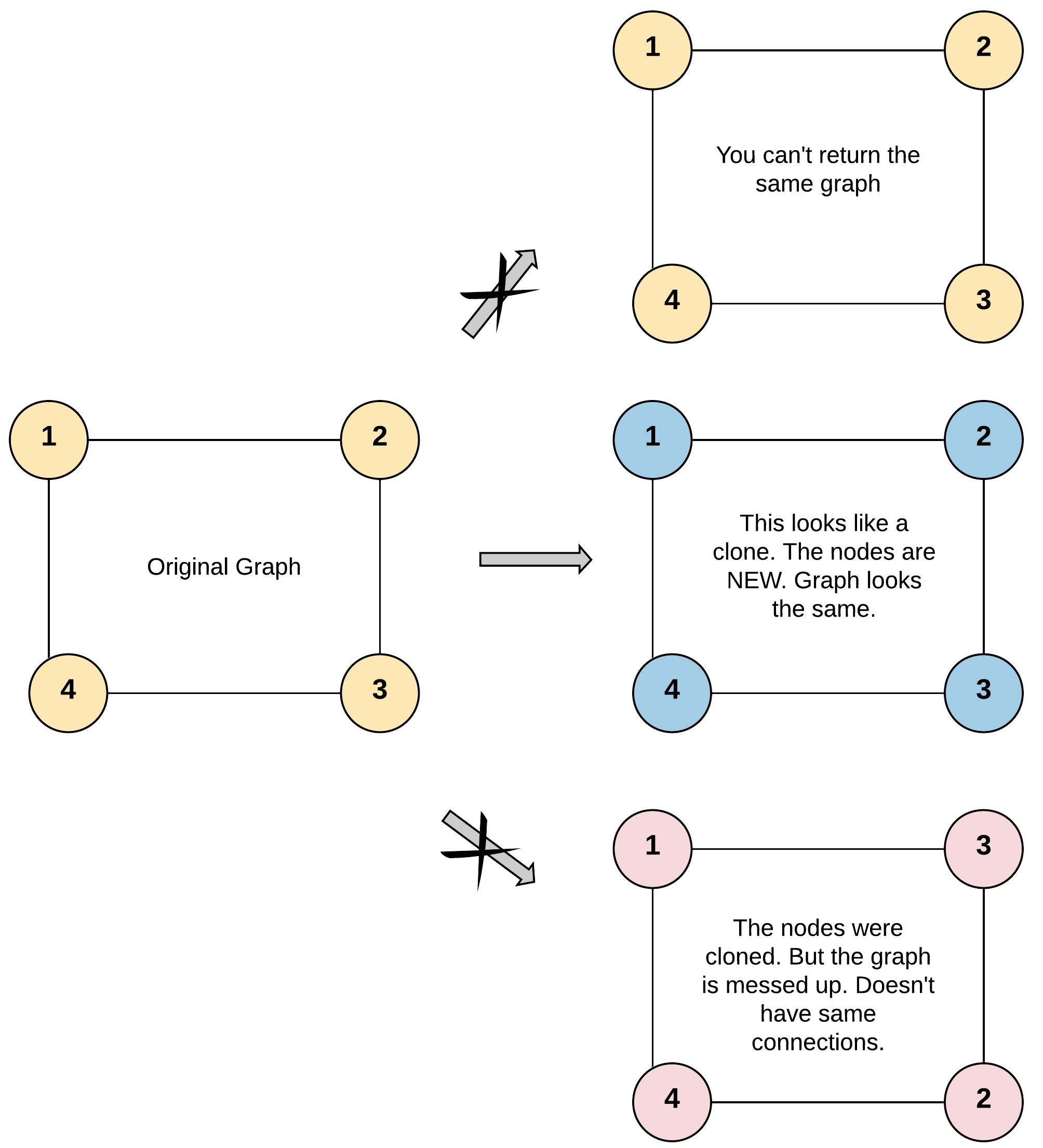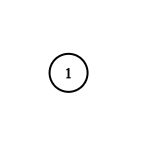## Algorithm

Problem Name: 133. Clone Graph

Given a reference of a node in a connected undirected graph.

Return a deep copy (clone) of the graph.

Each node in the graph contains a value (`int`) and a list (`List[Node]`) of its neighbors.

```class Node {
public int val;
public List<Node> neighbors;
}
```

Test case format:

For simplicity, each node's value is the same as the node's index (1-indexed). For example, the first node with `val == 1`, the second node with `val == 2`, and so on. The graph is represented in the test case using an adjacency list.

An adjacency list is a collection of unordered lists used to represent a finite graph. Each list describes the set of neighbors of a node in the graph.

The given node will always be the first node with `val = 1`. You must return the copy of the given node as a reference to the cloned graph.

Example 1:```Input: adjList = [[2,4],[1,3],[2,4],[1,3]]
Output: [[2,4],[1,3],[2,4],[1,3]]
Explanation: There are 4 nodes in the graph.
1st node (val = 1)'s neighbors are 2nd node (val = 2) and 4th node (val = 4).
2nd node (val = 2)'s neighbors are 1st node (val = 1) and 3rd node (val = 3).
3rd node (val = 3)'s neighbors are 2nd node (val = 2) and 4th node (val = 4).
4th node (val = 4)'s neighbors are 1st node (val = 1) and 3rd node (val = 3).
```

Example 2:```Input: adjList = [[]]
Output: [[]]
Explanation: Note that the input contains one empty list. The graph consists of only one node with val = 1 and it does not have any neighbors.
```

Example 3:

```Input: adjList = []
Output: []
Explanation: This an empty graph, it does not have any nodes.
```

Constraints:

• The number of nodes in the graph is in the range `[0, 100]`.
• `1 <= Node.val <= 100`
• `Node.val` is unique for each node.
• There are no repeated edges and no self-loops in the graph.
• The Graph is connected and all nodes can be visited starting from the given node.

## Code Examples

### #1 Code Example with C Programming

```Code - C Programming```

``````start coding...
struct UndirectedGraphNode *copy1node(struct UndirectedGraphNode *graph,
struct UndirectedGraphNode ***srcpool,
struct UndirectedGraphNode ***dstpool,
int *sp, int *sz) {
struct UndirectedGraphNode *node;
int i = 0;

if (!graph) return NULL;

while (i < *sp && (*srcpool)[i] != graph) {
i ++;
}
if (i == *sp) {
node = malloc(sizeof(struct UndirectedGraphNode));
//assert(node);
node->label = graph->label;
if (*sp == *sz) {
*sz = *sz * 2;
*srcpool = realloc(*srcpool, *sz * sizeof(struct UndirectedGraphNode *));
*dstpool = realloc(*dstpool, *sz * sizeof(struct UndirectedGraphNode *));
//assert(*srcpool && *dstpool && *stspool);
}
(*srcpool)[*sp] = graph;
(*dstpool)[*sp] = node;
(*sp) += 1;
i = 0;
while (i < graph->neighborsCount) {
node->neighbors[i] = copy1node(graph->neighbors[i], srcpool, dstpool, sp, sz);
i ++;
}
node->neighborsCount = graph->neighborsCount;
} else {
node = (*dstpool)[i];
}

return node;
}

struct UndirectedGraphNode *cloneGraph(struct UndirectedGraphNode *graph) {
struct UndirectedGraphNode **srcpool, **dstpool, *node;
int sp = 0, sz = 100;

srcpool = malloc(sz * sizeof(struct UndirectedGraphNode *));
dstpool = malloc(sz * sizeof(struct UndirectedGraphNode *));
//assert(srcpool && dstpool && stspool);

node = copy1node(graph, &srcpool, &dstpool, &sp, &sz);

free(srcpool); free(dstpool);

return node;
}
``````
Copy The Code &

Input

cmd

Output

cmd
[[2,4],[1,3],[2,4],[1,3]]

### #2 Code Example with C++ Programming

```Code - C++ Programming```

``````class Solution {
private:
unordered_mapm;
public:
UndirectedGraphNode *cloneGraph(UndirectedGraphNode *node) {
if(!node) return NULL;
if(m.count(node) == 0){
m[node] = new UndirectedGraphNode(node->label);
for(auto x: node->neighbors) m[node]->neighbors.push_back(cloneGraph(x));
}
return m[node];
}
};

// BFS
class Solution {
public:
UndirectedGraphNode *cloneGraph(UndirectedGraphNode *node) {
if(!node) return NULL;
unordered_mapm;
UndirectedGraphNode* root = new UndirectedGraphNode(node->label);
m[node] = root;
dequecur;
dequenext;
cur.push_back(node);
while(!cur.empty()){
UndirectedGraphNode* p = cur.front();
cur.pop_front();
for(auto x: p->neighbors){
if(m.count(x) == 0){
UndirectedGraphNode* copy = new UndirectedGraphNode(x->label);
m[x] = copy;
next.push_back(x);
}
m[p]->neighbors.push_back(m[x]);
}
if(cur.empty()) swap(cur, next);
}
return root;
}
};

``````
Copy The Code &

Input

cmd

Output

cmd
[[2,4],[1,3],[2,4],[1,3]]

### #3 Code Example with Java Programming

```Code - Java Programming```

``````
class Solution {
public Node cloneGraph(Node node) {
if (node == null) {
return null;
}
Map map = new HashMap<>();
map.put(node, new Node(node.val));
while (!queue.isEmpty()) {
Node removed = queue.remove();
for (Node neighbor : removed.neighbors) {
if (!map.containsKey(neighbor)) {
map.put(neighbor, new Node(neighbor.val));
}
}
}
return map.get(node);
}
}
``````
Copy The Code &

Input

cmd

Output

cmd
[[]]

### #4 Code Example with Javascript Programming

```Code - Javascript Programming```

``````
const cloneGraph = function(node) {
if (!node) return node
const map = {}
return traverse(node)
function traverse(node) {
if(!node) return node;
if (!map[node.val]) {
const newNode = new Node(node.val)
map[node.val] = newNode
newNode.neighbors = node.neighbors.map(traverse)
}
return map[node.val]
}
}
``````
Copy The Code &

Input

cmd

Output

cmd
[[]]

### #5 Code Example with Python Programming

```Code - Python Programming```

``````
class Solution:
def cloneGraph(self, node: "Node") -> "Node":
visited = {}

def dfs(node):
if node and node.val not in visited:
newNode = Node(node.val, [])
visited[newNode.val] = newNode
newNode.neighbors = [
visited.get(n.val) or dfs(n) for n in node.neighbors
]
return newNode

return dfs(node)
``````
Copy The Code &

Input

cmd

Output

cmd
[]

### #6 Code Example with C# Programming

```Code - C# Programming```

``````
using System.Collections.Generic;

namespace LeetCode
{
public class _0133_CloneGraph
{
public Node CloneGraph(Node node)
{
var map = new Dictionary();
return CloneGraph(node, map);
}

public Node CloneGraph(Node node, IDictionary map)
{
if (node == null) return null;
if (map.ContainsKey(node.val)) return map[node.val];

var newNode = new Node(node.val, new List());
foreach (var child in node.neighbors)

return newNode;
}

public class Node
{
public int val;
public IList neighbors;

public Node() { }

public Node(int _val, IList _neighbors)
{
val = _val;
neighbors = _neighbors;
}
}
}
}
``````
Copy The Code &

Input

cmd